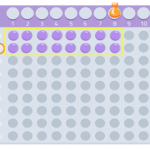Smartick is an advanced online program that teaches kids math and coding in only 15 min. a day

Jul21

## Divisibility Criteria for 3, 4, 9 and 11In this post we are going to learn about the criteria of divisibility by 3, 4, 9 and 11. Criteria of divisibility by 3 A number is divisible by 3 when the sum of its digits is a multiple of 3. For example: Is 1098 divisible by 3? We add all the digits of 1098: 1 […]

Mar09

## Reducible Equations: What Are They? How Do We Solve Them?In this post, we are going to explain reducible equations with several examples taken from Smartick’s daily sessions. We will begin with some easier examples, and work our way toward those that are more complicated. If you need to review what an equation is, and its terms, you can take a look at this previous […]

Sep02

## What is Factorial Decomposition?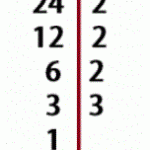In today’s post we are going to answer the question, what is factorial decomposition? I hope that by the end of the post you’ll have it crystal clear. Definition of Factorial Decomposition We are going to start by remembering what a ”factor” is in mathematics. A factor is every number that is multiplied by another. […]

Dec24

## Some Prime Number Curiosities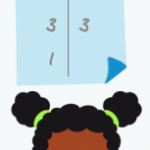For this entry, we are going to learn some surprising facts about prime numbers. We would recommend reading our post about prime numbers and composite numbers first, to refresh your knowledge about these numbers. Are you ready to move on? Perfect! I’m sure that you know it is possible to write some numbers as products of […]

Jul23

## Explanation of the Formula to Calculate the Least Common Multiple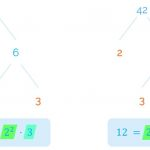I am sure this sounds familiar to many of you: To calculate the least common multiple (LCM), we have to choose between the common and uncommon factors that have the highest exponent. But what does it mean? Why do we have to choose from all factors…and out of the common factors, why the ones with […]

Mar12

## Using the LCM (Least Common Multiple) to Solve Problems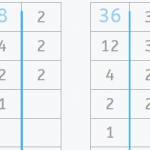In a previous post, we looked at the Least Common Multiple and how to calculate it. Today we’re going to look at a couple of examples in which we use the Least Common Multiple (LCM) to solve math problems. In one example, we’ll use the LCM to calculate the answer directly, and in the other we’ll […]

Feb02

## Prime Numbers Activities with Smartick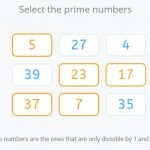In previous posts we’ve learned about prime numbers and composite numbers. Today we’re going to look at different sequenced prime numbers activities involving prime numbers that Smartick students work on. We’ve included examples of different types and levels, from identifying whether a number is prime or composite to finding the least common multiple and highest common factor through […]

Nov27

## Prime Numbers: How to Find Them with the Sieve of Eratosthenes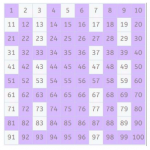In today’s post, we’re going to learn how to find prime numbers using the Sieve of Eratosthenes. A prime number is one which is only divisible by 1 and itself. It’s as simple as that; the downside is that there’s no mathematical formula to make sure whether a number is prime or not. Think of a high number like 191,587. […]